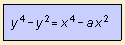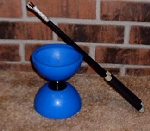# devil's curve

## quarticThis devil's curve is also known as the devil on two sticks. Gabriel Cramer (1704 - 1752) was the first to investigate the curve, in 1750 1). Cramer was a Swiss mathematician, he is best known for his work on determinants.Lacroix 2) studied the curve in 1810. And there was a publication about the curve in the Nouvelles Annales de Mathťmatiques in 1858 3). Many authors place an extra constant before the y2 term, but this is only a linear distortion of the curve.It seems to me that the devil in the name of the curve is from the diabolo game, being the form of the curve. The confusion is the result of the Italian word diabolo meaning 'devil'. This makes also clear the meaning of the sticks in 'the devil on two sticks', these are the sticks used to handle the diabolo. For a=25/24 the curve is called the electric motor curve. The middle of the curve shows the coils of a wire, which rotate by means of the forces exerted by the magnets around. The double devil's curve results when combining the curves for a and 1/a. notes 1) Introduction a l'analyse des lignes courbes algťbriques, p. 19 (Genova, 1750). Cramer mentionned as equation: y4 - 96 y2 - x4 + 100 x2 = 0, so that a = 100 / 962. 2) Traitť du calcul diffťrentiel et du calcul intťgral volume I, 334-336 (Paris, 1797; 1810 ed.). Lacroix introduced a parameter a, in equation: y4 - 96 a2 y2 + 100 a2 x2 - x4 = 0, so that a = a(Lacroix) * 100 / 962. 3) Page 317 4) La courbe du diable, the American mathematical monthly, vol. 33, p.273